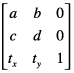In previous part, we talked about 4 properties of `UIView` and relationships between them. This part we cover operations with `transform` and how to persist `UIView` information. Let’s go!

## `transform` operations

`transform` is type of `CGAffineTransform` and it represents a matrix used for affine transformation. Speaking of the matrix and and affine transformation, it is beyond context of this article, but if you wonder and want to learn more, this wiki would be a good start.

In brief, `transform` includes 6 matter values `a`, `b`, `c`, `d`, `x` and `y` as the figure below.`a` and `d` are for scale, `x` and `y` are for translation and `a`, `b`, `c` and `d` are for rotation. However, usually, we use only scale and rotation of `transform` as for translation, we can simply use `center` property to position a view in its superview.

When `transform` is used for only one transformation, it is straightforward to extract that information, e.g. factor of scale or angle of rotation. In most of cases, however, we use combination of scale and rotation, that makes transformation extraction more tangled. Thanks to mathematics, we already have equations for this and only need to convert to code.

#### Extract

Right below is snippet for scale extraction

``````func scaleOf(transform: CGAffineTransform) -> CGPoint {
let xScale = sqrt(transform.a * transform.a + transform.c * transform.c)
let yScale = sqrt(transform.b * transform.b + transform.d * transform.d)

return CGPoint(x: xScale, y: yScale)
}
``````

and for rotation.

``````func rotationOf(transform: CGAffineTransform) -> CGFloat {
return CGFloat(atan2(transform.b, transform.a))
}
``````

#### Restore

In reverse, `CoreGraphics` provides handy functions to create `CGAffineTransform` from transformation information.

``````view.transform = CGAffineTransformMakeRotation(angle_in_radian) // Create CGAffineTransform of rotation transformation

view.transform = CGAffineTransformMakeScale(xScale, yScale) // Create CGAffineTransform of scale transformation
``````

Because order of transformation matters, in case of combination of scale and rotation, we should persist the whole `transform`, i.e., `a`, `b`, `c`, `d`, `x` and `y` values, and use it to restore itself.

``````view.transform = CGAffineTransform(a: a, b: b, c: c, d: d, tx: x, ty: y);
``````

## Persistence

When comes with `UIView` information persistence, especially with `transform` is applied, note following things:

• `frame` is invalid so we shouldn’t use it to position or measure view.
• Though `frame` is invalid, there is way to calculate actual values and use them for positioning or sizing of view.
• `center` is recommended to position view.
• `bounds.size` is recommended to measure view.

So what information we need to persist? They are position in superview, size and transformation. With transformation, we can persist `transform` as a whole or individual one.

#### Position

The best way is to persist `center`, as `center` is independent of `transform`, it is safe to position view regardless of `transform`.

``````let persistedCenter = view.center
``````

However, in some scenarios, `frame.origin` may be useful, then we need calculation on restoration to convert `frame.origin` back to `center`. It will be there later.

``````let persistedPosition = frame.origin
``````

#### Size

Both `bounds.size` and `frame.size` cannot be used for size persistence, because they do not reflect correct view’s size. `bounds.size` is original value before transformation. And `frame.size` is size of boundary view, not actual view.

So how? The answer is to use `bounds.size` and scale transformation and it is pretty simple.

``````let scale = scaleOf(view.transform)
let persistedSize = CGSize(width: view.bounds.size.width * scale.x, height: view.bounds.size.height * scale.y)
``````

If we count actual view’s size in persistence, `transform` we persist should contain only rotation transformation. This is due of restore phrase later. Otherwise, restoration will get unnecessary calculation, now or later is of your choice. Then I choose now and it also makes more sense to persist real view’s size than its original one.

#### Transformation

How to persist position and size affects how to do with transformation, actually, only size does. As stated right above, if we persist actual view’s size, rotation is the only thing we need for transformation.

``````let persistedRotation = rotationOf(view.transform)
``````

How about original view’s size? So we have to persist the whole `transform` by using its values.

``````let a = view.transform.a
let b = view.transform.b
let c = view.transform.c
let d = view.transform.d
``````

With these values, it is easy to restore `transform` as talked above.

#### Restore

Make assumption that we are persisting actual view’s size, its rotation and `center`, now it is time to restore the view from that.

``````let view = UIView(frame: CGRect(x: 0, y: 0, width: persistedSize.width, height: persistedSize.height))
view.transform = CGAffineTransformMakeRotation(persistedRotation)
view.center = persistedCenter
``````

How about `frame.origin` for position? Here we are.

``````let view = UIView(frame: CGRect(x: 0, y: 0, width: persistedSize.width, height: persistedSize.height))
view.transform = CGAffineTransformMakeRotation(persistedRotation)
view.center = CGPoint(x: persistedPosition.x + view.frame.size.width / 2, y: persistedPosition.y + view.frame.size.height / 2)
``````

## Conclusion

There are still many more to talk about `UIView`, however, with this article, `transform` property is not scary anymore, huh? From here, we can keep learning `layer.anchorPoint` which is also relevant to `transform`, animation with `transform` and other 3 properties, etc. Happy learning!